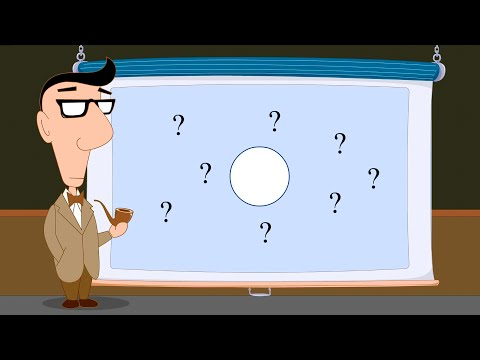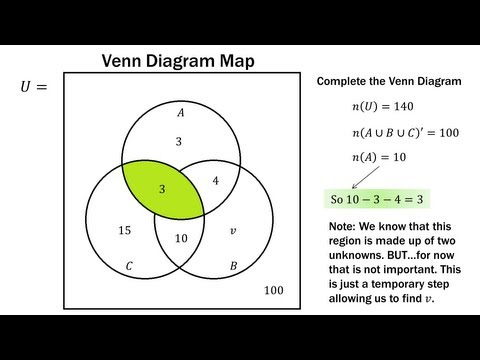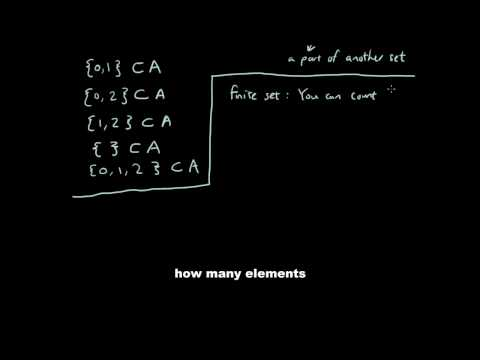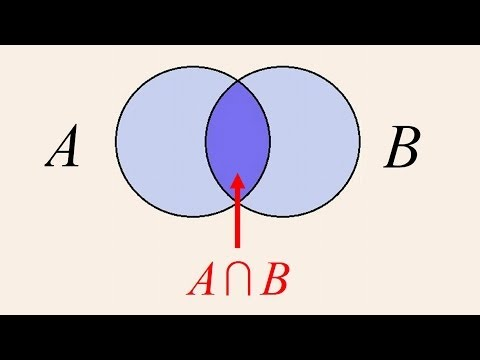## Introduction to Sets

Subject: Compulsory Mathematics#### Overview

In the early twentieth century, John-Euler Venn solved the word problems in arithmetic with the help of Venn-diagram. This method grew popular as it is easy to understand and simple to calculate. So, Venn-diagram is associated with his name. There are 6 types of sets, they are as follow: Empty set, Singleton set, Finite set, Infinite set, Equivalent set & Equal set.
##### Introduction to Sets

In the early twentieth century, John-Euler Venn solved the word problems in arithmetic with the help of Venn-diagram. This method grew popular as it is easy to understand and simple to calculate. So, Venn-diagram is associated with his name.

### Types of sets

#### Empty/Null set

A set which does not contain any element is called an empty set or null set. It is denoted by $\emptyset$
empty, and is read as phi. In roster form, $\emptyset$ it is denoted by { }.
For example:

1. The set of the whole number less than zero. Clearly there is no whole number less than zero.
Therefore, it is an empty set.
2. Let A = {x:2 < x <3, x is a natural number}
Here A is an empty set because there is no natural number between 2 and 3.
 Note $\emptyset$ ≠ { 0 } { 0 } is a set having one element.

#### Singleton set

A set which has only one element is called singleton set.
For example:1. A = { x:x neither prime nor composite}
It is a singleton set only containing one element i.e. 1.
2. Let B = { x:x $\in$ N and x2 = 4}
It is a singleton set only containing one element i.e. 2, which square is 4.

#### Finite set

A set which contains a definite number of elements is called a finite set. The empty set is also called a finite set.
For example:

1. The set of all color in the rainbow.
2. A = { 1, 2, 3, 4, 5, 6, 7, 8, 9, ........................................ 50 }

#### Infinite set

A set which contains never ending element is called infinite set.
For example:

1. Set of all point in the plain.
2. Set of all prime number.
3. B = { x:x $\in$ W, x = 2n }

#### Cardinal number of set

The number of distinct element in a given set A is called the cardinal number of A. It is denoted by n(A).
For example:

• B = { x:x $\in$ N, x<5}
B = {1,2, 3, 4}

#### Equivalent set

Two set are called to be equivalent if their cardinal number is same i.e. n(A)=n(B). The symbol for denoting an equivalent set is '$\leftrightarrow$'.
For example:

A = {1, 2, 3, 4, 5}
B={a, b, c, d, e }
Therefore, A $\leftrightarrow$ B.

#### Equal set

Two set are said to be equal if they contain the same element. Every element of a set A has every element of B and every element of B has every element of A.
For example:

A= { a,e, i,o, u }
B= {u, i, o, a, e}

$\therefore$ A = B

##### Things to remember
1. n(A∪B∪C) = n(A) + n(B) + n(C) - n(A∩B) - n(C∩A) - n(B∩C) + n(ABC)
2. Maximum value of n(A∩B) ↔ minimum value of n(A∩B)
3. Minimum value of n(A∩B) ↔ Maximum value of n(A∩B)
4. The cardinality of a set is a positive integer but it is not decimal. So n(A)  ≠ 50% because 50% = 0.5
5. Total of cardinality shown in Venn-diagram must be equal to the cardinality of ∪.

• It includes every relationship which established among the people.
• There can be more than one community in a society. Community smaller than society.
• It is a network of social relationships which cannot see or touched.
• common interests and common objectives are not necessary for society.
##### Videos for Introduction to Sets##### Complement and Relative Complement##### Finite Math: Venn Diagram Practice Problems##### Set: Explanation and Type 1 Question##### Subset, Empty set, Finite/Infinite set, Equal/Equivalent set